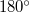﻿

### On a paper of Dan Barbilian

#### Abstract

En
We point out that the axiomatic analysis of the statement The segments joining a point with the vertices of an equilateral triangle satisfy the (non-strict) triangle inequalities in Barbilian’s  misses the case in which the sum of the angles in a triangle is greater than$180^{\circ}$.
We situate the statement correctly inside absolute geometry. We also point out that  contains the first proof that a Hilbert geometry with symmetric perpendicularity must be hyperbolic geometry, a proof commonly attributed to P. J. Kelly and L. J. Paige .

DOI Code: 10.1285/i15900932v29n2p29

Keywords:
Hilbert geometry; the Mòbius-Pompeiu inequality; absolute geometry

Full Text: PDF